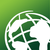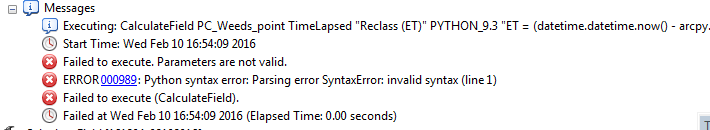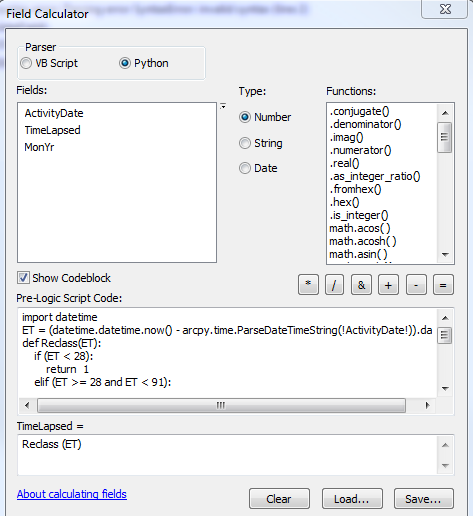# Calculate field conditional statement using a date formula

5808
11
02-09-2016 10:25 PMNew Contributor II

Hi all,

I am using ArcGIS 10.3 to update a field with a numeric value that represents the elapsed time since the data was captured.  I can do this in a python script that I have attached to a model but I also want to be able to sometimes do it quickly from within ArcGIS desktop and I am stumped.

I have written a 3 step process:

1. Create an integer field then calculate the number of days since today (field name = ET):

In Field Calculator, ET =

(datetime.datetime.now() - arcpy.time.ParseDateTimeString(!ActivityDate!)).days

2.  Create a conditional Statement for "Elapsed Time" field as follows:

Expression:

Reclass(!ET!)

def Reclass(ET):

if ( ET < 28):

return 1

elif ( ET>= 28 and ET < 91):

return 2

elif ( ET >= 91 and ET < 182):

return 3

elif ( ET >= 182 and ET < 365):

return 4

elif (ET >= 365):

return 5

3. Delete the ET field

I really just want to declare a variable in my conditional statement for ET which would save having to create then delete the ET field, but everything I have tried does not work, for example:

Expression:

Reclass(ET)

ET = (datetime.datetime.now() - arcpy.time.ParseDateTimeString(!ActivityDate!)).days

def Reclass(ET):

if ( ET < 28):

return 1

etc etc

I cannot find any examples of conditional statements that use a variable instead of a field value in the Field Calculator, so any help would be appreciated.

1 Solution

Accepted SolutionsNew Contributor II

OK thanks Dan! Below is what worked.  (It didnt work if I parsed the ET function into nowww before the def block though).

Expression:

Reclass(!ActivityDate!)

import datetime

def Reclass(DateFld):

ET = (datetime.datetime.now() - arcpy.time.ParseDateTimeString(DateFld)).days

if (ET < 28):

return  1

elif (ET >= 28 and ET < 91):

return 2

elif (ET >= 91 and ET < 182):

return 3

elif (ET >= 182 and ET < 365):

return 4

elif (ET >= 365):

return 5

11 RepliesbyMVP Frequent Contributor

And what errors are you getting?

Have you imported the datetime module?New Contributor II

The error says Error 000989: Python syntax error: Parsing error SyntaxError: invalid syntax (line 1).

I dont import the datetime module in Field Calculator.

The code for calculating the difference between today and and the collection day as an integer value works fine in Field calculator  but its not happy when you try to create a variable using the same code.byMVP Esteemed Contributor

you need to import datetime... just put it in front of the def linebyEsri Esteemed Contributor

... and if it is not the missing import statement, you may want to look at the content of the field !ActivityDate!. If the content is not valid and cannot be parsed to a datetime it will fail at arcpy.time.ParseDateTimeString(!ActivityDate!)New Contributor II

Unfortunately the import datetime does not work - I'm still getting the same error.

just to re-iterate, I am using arcGIS 10.3 desktop and am using the Calculate Field tool.

The code now looks like this (but doesn't work):

import datetime

ET = (datetime.datetime.now() - arcpy.time.ParseDateTimeString(!ActivityDate!)).days

def Reclass(ET):

if (ET < 28):

return  1

elif (ET >= 28 and ET < 91):

return 2

elif (ET >= 91 and ET < 182):

return 3

elif (ET >= 182 and ET < 365):

return 4

elif (ET >= 365):

return 5

!ActivityDate! content does contain valid date values and the code string

(datetime.datetime.now() - arcpy.time.ParseDateTimeString(!ActivityDate!)).days

works fine on its own, just wont work when I use it in this context.byMVP Esteemed Contributor

ET should be inside the def, just after it

def Reclass(a_fld)

ET = blah blah blah ...TimeString( a_fld )

Reclass(!ActivityDate! ) should be in the timelapesed = box

You pass a field to the function

The function will contain a variable name, it shouldn't be the actual field name

your ET function should be within the def block not before

You should really parse the ET function into

nowww = (datetime.datetime.now()   # before the code block

ET = (nowww - the reset of the original equation)New Contributor II

OK thanks will try thatNew Contributor II

OK thanks Dan! Below is what worked.  (It didnt work if I parsed the ET function into nowww before the def block though).

Expression:

Reclass(!ActivityDate!)

import datetime

def Reclass(DateFld):

ET = (datetime.datetime.now() - arcpy.time.ParseDateTimeString(DateFld)).days

if (ET < 28):

return  1

elif (ET >= 28 and ET < 91):

return 2

elif (ET >= 91 and ET < 182):

return 3

elif (ET >= 182 and ET < 365):

return 4

elif (ET >= 365):

return 5New Contributor II

PS I just tried it without import datetime and it still works (which makes sense)